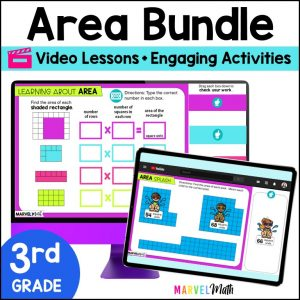Save 10% on your first order with code EXTRA10

# Area of Rectangles using Side Lengths – Digital Activities + Video Lesson

\$4.00\$16.80
SKU: 5468194 Categories: ,

## Description

Your students will love the bright and engaging Google Slides Activities to practice finding the Area of Rectangles. You will love the ready-made Video Lesson. This resource is perfect for your lesson, math stations, review or tutoring. Students will learn how to calculate the area by multiplying whole number side lengths. This Simple and Easy-to-Use lesson is designed for 3rd or 4th grade students and aligns to CCSS 3.MD.C.7b and TEKS 4.5D

What Teachers are Saying:

⭐⭐⭐⭐⭐ This resource was so engaging and my students loved it! -Kayleigh H.

⭐⭐⭐⭐⭐ I really loved this resource! It was a perfect review for what my students were struggling with! Thank you! -Tasha L.

The Video Lesson:

• Teaches students how to multiply side lengths of rectangles to find the area
• Show students how to use the formula A = l x w
• Walks students through the Google Slides activities they will complete
• Is approximately 4 minutes
• Student Devices must have access to You-Tube to view the Video Lesson

⭐Many of the slides are self-checking, so students can work through the activity with confidence.

Activities Include:

• Engaging practice with Movable Pieces
• Matching Activities
• Application of Learning in Real-World Word Problems
• Word Problems have optional Step-by-Step support

What’s Included?

• Video Lesson with Activity Instructions
• 11 Interactive Activities
• Teacher Key in separate Google Slides™ doc that can be assigned to students to check their work at home with their parents
• Directions on how to assign the Google Slides™ activity to students in Google Classroom™

Standards Alignment

CCSS.3.MD.C.7b Multiply side lengths to find area of rectangles with whole-number side lengths in the context of solving real world and mathematical problems, and represent whole-number products as rectangular areas in mathematical reasoning.

TEKS 4.5D Solve problems related to perimeter and area of rectangles where dimensions are whole numbers

This is Lesson 3 in a comprehensive bundle that covers all the area topics your students need to learn!

Save 30% on this resource by purchasing the Digital Area Bundle!

Lessons in the Digital Area Bundle Include:

Area Lesson 1: Understand the Concept of Area by filling Rectangles with Square Units

Area Lesson 2: Find Area by Multiplying the Number of Rows by the number of Unit Squares in Each Row

Area Lesson 3 *This Resource*: Multiplying Whole Number Side Lengths to find Area

Area Lesson 4: Area of Irregular Shapes using Unit Squares

Area Lesson 5: Area of Irregular Shapes using Side Lengths

Area Lesson 6: Use Area Models to Prove the Distributive Property